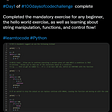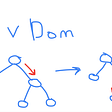# Math is fun

Straight forward

`class Solution:    def sumFourDivisors(self, nums: List[int]) -> int:        res = 0        for n in nums:            divisors = {1,n}            for k in range(2, int(n**0.5)+1):                if n%k==0:                    divisors.add(k)                    divisors.add(n//k)                if len(divisors)>4:break            else:                if len(divisors)==4:res+=sum(divisors)        return res`

By using the Sieve of Eratosthenes algorithm to list all the prime numbers between 2 to N.

Since the Sieve of Eratosthenes algorithm has a complexity of O(n), this solutions has the complexity of O(N+M+K²) where N = max(nums), M=len(nums), K is len(primes)

then we can get all the numbers which have exactly 4 divisors. they are

case a: 1, p,p*p, p*p*p

case b:1, p, q, p*q

`class Solution:    def sumFourDivisors(self, nums: List[int]) -> int:        N = max(nums)        four_div = [False] * (N + 1)        #four_distinct_factors_sums        sums = *(N+1)                def sieve_of_eratosthenes():            is_prime = [True] * (N + 1)            p = 2             while p * p <= N:                  if is_prime[p]:                     i = p + p                     while i <= N:                         is_prime[i] = False                        i += p                p += 1            return [p for p in range(2, N+1) if is_prime[p]]           def update_four_distinct_factors_sums():                       primes = sieve_of_eratosthenes()            for i, p in enumerate(primes):                 #case a: 1, p,p*p, p*p*p                case1 = p**3                if (case1 <= N):                     four_div[case1] = True;                     sums[case1] = 1+p+p**2+case1                #case b:1, p, q, p*q                for j in range(i + 1, len(primes)):                     q = primes[j]                    case2 = p*q                    if (case2 > N):break                    four_div[case2] = True                    sums[case2] = 1+p+q+case2        update_four_distinct_factors_sums()        return sum(sums[n] for n in nums if four_div[n])`

--

--

--

## More from Jimmy Shen

Data Scientist/MLE/SWE @takemobi

Love podcasts or audiobooks? Learn on the go with our new app.

## Data Vault Advent Calendar 10/25## Coding: My Journey So Far## Announcing ArthSwap IDO Launchpad Pre-Release Campaign!## Programming Is A Metaphor Of Life. Just Like Football## What is Web API Security❓ — Methods of Protection## Anoma Research and Development Update: April 2022## Jimmy Shen

Data Scientist/MLE/SWE @takemobi

## A Trip to Space: Implementing the Machine Learning Algorithm of Spectral Clustering## “C’est la vie”, or how I feel about my first job.## Prepare for React interview: Reactivity and virtual DOM